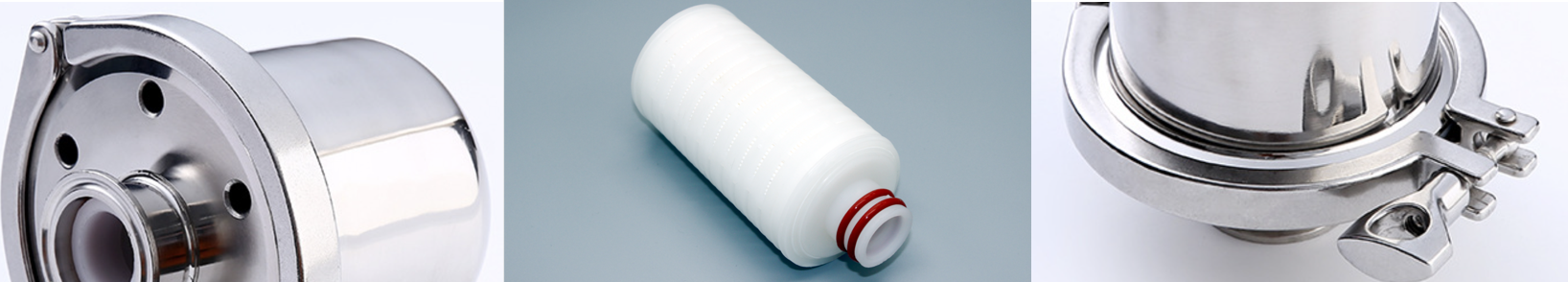# 公司产品# 气体除菌过滤器-呼吸器

• 产品概述
• 规格参数
• 产品选型0.10μm≥0.12Mpa   0.22μm≥0.09 Mpa   0.45μm≥0.06 MpaHX4050PF0022S 产品系列代码 壳体材质 壳体长度 滤芯材质 过滤精度 密封材质 HX 4=SUS304不锈钢） 025=2.5英寸 PF=疏水PTFE 0001=0.01μm S=硅橡胶 6=SUS316不锈钢 050=5英寸 PV=疏水PTFE 0010=-0.1μm M=三元乙丙 PP=PP聚丙烯 0022=0.22μm N=丁腈橡胶 JS=不锈钢滤芯 0045=0.45μm V=氟橡胶 0100=1μm（PP,JS） T=铁氟龙包氟 0500=5μm（PP,JS） 1000=10μm（PP,JS） 5000=50μm（PP,JS）

# 相关推荐X